# Activation Functions in Neural Networks

The activation function is a function used in neural networks to calculate the weighted sum of inputs and biases, which is used to determine whether a neuron should be activated or not. You must have heard a lot about activation functions while studying machine learning, deep learning, or neural networks. If you don’t know what activation functions are, this article is for you. In this article, I will give you an introduction to activation functions in neural networks and the types of activation functions you should know.

# Activation Functions

Activation Functions are functions used in neural networks for calculating the weighted sum of input and biases. It’s done to determine if a neuron should be activated or not. What happens is that the input layer receives the training data for training the neural network, the hidden layer is used to do all the calculations which help in finding relationships between the features of the data, and then the output layer gives the results which are controlled by the activation functions.

The position of activation functions in neural network architectures depends on their functions. The activation functions are placed after the hidden layers to produce the results for the neural network. I hope you now have understood the need for activation function in a neural network. Different types of activation functions are used in neural network architectures. In the section below, we’ll explore the types of activation functions in neural networks that you should know.

# Types of Activation Functions

## Sigmoid Function

The sigmoid activation function is a nonlinear activation function commonly used in feedforward neural networks. They are used to train neural network architectures to predict probability. Thus, a sigmoid activation function can be used for binary classification problems.

## Hyperbolic Tangent Function

Another type of activation function used in neural networks is the hyperbolic tangent function. It is also known as the tanh function. It is commonly used in recurrent neural network architectures to solve problems based on natural language processing.

## Softmax Function

The softmax function is a popular activation function used in neural network architectures. Like the sigmoid activation function, the softmax function is also used in training models to predict probability. The difference is that the sigmoid function is used in binary classification problems, and the softmax function is used in multiclass classification problems.

## Rectified Linear Unit Function (ReLU)

The rectified linear unit or ReLU is the most widely used activation function in neural network architectures. It is a faster activation function and has better performance and generalization compared to all other activation functions. The ReLU activation function is widely used in deep neural network architectures to solve problems such as object recognition and speech recognition.

# Summary

Activation Functions are functions used in neural networks for calculating the weighted sum of input and biases. It’s done to determine if a neuron should be activated or not. I hope you now have understood what is an activation function and what are the types of activation functions. There are more types of activation functions, but they are not commonly used in neural network architectures. You can explore more about them from here. I hope you liked this article on activation functions and their types. Feel free to ask your valuable questions in the comments section below.

## More from Aman Kharwal

I write stories behind the data📈 | https://www.instagram.com/the.clever.programmer/

Love podcasts or audiobooks? Learn on the go with our new app.

## Automatic vehicle number plate detection using YOLOv5 and extraction of text using OCR## FEDERATED LEARNING: THE FUTURE OF DISTRIBUTED MACHINE LEARNING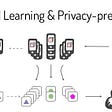## Building a Quick and Simple Insult Classifier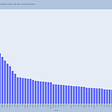## Reading: VRCNN-BN — Variable-filter-size Residue-learning Convolutional Neural Network with Batch…## The Bare Basic Breakdown of Machine Learning## Support Vector Regression## Hyperelastic material model Arruda-Boyce for Nonlinear Finite Element Analysis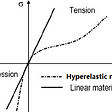## Named Entity Extraction In Legal Documents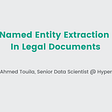## Aman Kharwal

I write stories behind the data📈 | https://www.instagram.com/the.clever.programmer/

## Keras Tutorial for Beginners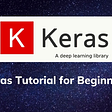## Parametric and Nonparametric Machine Learning Algorithm## What is a Neural Netwok? Start your Deep Learning Journey today !!!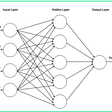## The science behind Ensemble Learning